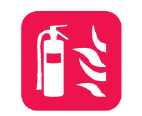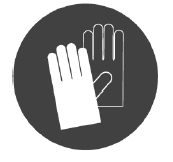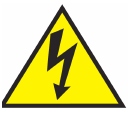# Mock exam paper C Higher Level Marking Scheme

PRE-LEAVING CERTIFICATE EXAMINATION 2012

ENGINEERING – MATERIALS AND TECHNOLOGY

(Higher Level – 300 marks)

Duration: 3 hours

W: http://www.irevise.com/ T: 091 - 520400INSTRUCTIONS

1. Answer Sections A and B of Question 1 and FOUR other questions.

2. All answers must be written in ink on the answer book supplied.

3. Diagrams should be drawn in pencil.

4. Squared paper is supplied for diagrams and graphs as required.

5. Please label and number carefully each question attempted.

LEAVING CERTIFICATE ENGINEERING

MATERIALS AND TECHNOLOGY

(Higher Level – 300 marks)

Marking Scheme 2012

Answer Question 1, Sections A and B and Four other questions.

Question 1 Section A – 50 marks- Any ten @ 5 marks each.

(a) 3 + 2

(b) 5

(c) 5

(d) Any two @ 3 + 2

(e) 3 + 2

(f) 3 + 2

(g) 3 + 2

(h) Any two @ 3 + 2

(i) 5

(j) 5

(k) 5

(l) 5

(m) 3 + 2

Question 1 Section B – 50 marks

(n) 2+2+2+2+2

(o) Any three at 3+3+3+1

(p) three @ 3 + 1

(q) (i) 5

(ii) 5

(r) 3+3+3+1

Question 2 – 50 marks

(a) Any two @ 8 + 8

(i) 5

(ii) 5

(c) (i) 6

(ii) Describe 5

Diagram 5

Question 3 – 50 marks

(a) Any two @ 8 + 8

(b) (i) 14: (7 Regions @ 2 marks each)

(ii) 2 + 2

(c) (i) 4

(ii) 12

Question 4 – 50 marks

(a) Any two @ 8 + 8

(b) (i) 4

(ii) 2 + 2 + 2 + 2 + 2

(iii) 4

(c) (i) 4 + 4

(ii) 8

Question 5 – 50 marks

(a) (i) 4

(ii) 10

(iii) 4

(b) Any three @ 5 + 5 + 5

(c) Any one @ Describe 8

Diagram 8

OR (c) (i) 4 + 4

(ii) 4 + 4

Question 6 – 50 marks

(a) (i) Name 2

Operation 6

(ii) 2 + 2 + 2

(iii) 2

(b) Any three @ 6 + 6 + 6

(c) (i) 5

(ii) 5

(iii) 3 + 3

Page 4 of 24

Question 7 – 50 marks

(a) Any three @ 6 + 6 + 6

(b) (i) 8

(ii) 4 + 4

(c) Any one @ 16

OR

(c) (i) 4

(ii) 4 + 4 + 4

Question 8 – 50 marks

(a) Any one @ Name 8

Application 8

(b) Any three @ 6 + 6 + 6

(c) Describe 8

Diagram 8

OR

(c) (i) Identify 2 + 2 + 2 + 2

(ii) Operation 4

Application 4

QUESTION 1. (100 MARKS)

SECTION A

Give brief answers to any ten of the following:

1. State the purpose of any two of the safety symbols shown.• Hazard or warning of electrical danger
• Always wear protective clo...